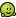Vanilla 1 is no longer supported or maintained. If you need a copy, you can get it here.

# Change style based on time/date?

I'd like to automagically switch to a darker theme at night. Is this possible?

• Bump.. I posted this a couple of months ago, perhaps someone has an idea of how to achieve this. After this bump I will leave it alone``` function check_time(&\$Context) { global \$compare_time; \$compare_time = ""; if (file_exists(\$Context->Dictionary["GRAPH_FILE"])) { \$compare_time = date ("Y-m-d H:i:s", filemtime(\$Context->Dictionary["GRAPH_FILE"])); list(\$year, \$month, \$day, \$hour, \$minute, \$second ) = sscanf(\$compare_time, "%d-%d-%d %d:%d:%d"); \$thatdate = mktime( \$hour, \$minute, \$second, \$month, \$day, \$year ); \$now = date("Y-m-d H:i:s",time()); // echo \$now."-".TimeAgo(\$thatdate); \$timeparts = explode(' ',\$compare_time); \$strt = \$timeparts; \$starttime = \$strt.substr(\$timeparts,1); \$timeparts = explode(' ',\$now); \$endt = \$timeparts; \$endtime = \$endt.substr(\$timeparts,1); \$diff = \$endtime - \$starttime; // echo \$strt."-".\$endt."--- ".\$diff." ***"; if (\$diff > \$Context->Dictionary["GRAPH_TIME"]) return true; return false; } return false; } ```